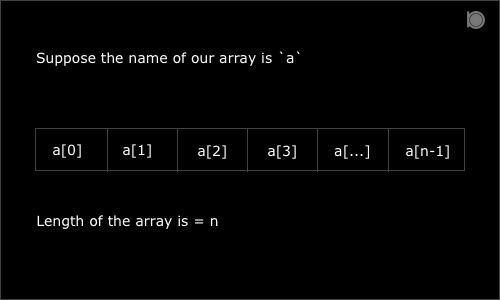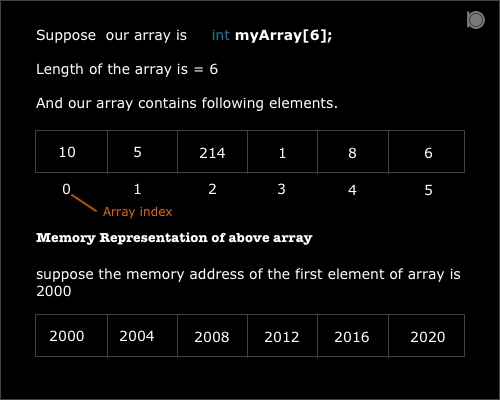Some time we need to group some similar data together to perform certain action on them and this is possible in C language because of an array.

Array is the collection of homogeneous data types. Instead of declaring each elements of a group individually, with the help of array we can declare the whole collection with single under single name.In the above picture `a`, `a`.. so on represents the array elements. And `0`, `1`, `2`, `3``n-1` represents the array index.

## Types of array

1. single dimensional arrays
2. multi-dimensional arrays

## Declaring an Array

To declare an array we need to specify the name, type and size of an array.

##### Syntax for declaring an array
``````type array_name[ length ];
``````

The above syntax is of single dimensional array. Here type is the data type of array, array_name is name of an array and length is the number of elements that array can hold.

###### Example

Suppose we want an array of integers having 5 elements. Let us name our array as `num`. Below is the example how we declare our array.

``````int num;
``````

## Initializing the Array

For initializing the array we need to provide array elements. There are different methods of initializing our array like to initialize all array elements at same time or to initialize each element individually.

##### Syntax for initializing the array
``````type array_name[ length ] = { element1, element2,.... };
``````
###### Example
``````int myArray = { 10, 5, 214, 1, 8, 6 };
``````

#### OR we can omit the array length

``````int myArray[] = { 10, 5, 214, 1, 8, 6 };
``````

#### OR we can initialize each element individually

``````myArray = 10;
myArray = 5;
myArray = 214;
myArray = 1;
myArray = 8;
myArray = 6;
``````

While initializing individual elements we need to provide array index or position of element in array. The array index starts from 0 for first element and the last index will be n-1 where n is the length of array.## Accessing or Reading Array elements

To read or access the array elements we simply need to provide the position or index of the element in the array.

##### Syntax for accessing array elements
``````array_name[ index ];
``````
###### Example
``````int num = myArray;
``````

## Creating and Reading an array using loop

The easiest and the fastest way to create new array or read a bunch of elements from array is by using loop.

In the following example we will use for loop to insert and read elements from an array.

### Program to insert and read elements in an array using for loop

``````//An example of creating and reading array using the for loop
#include<stdio.h>

int main()
{
int array;
int i;

for(i=0; i<10; i++)
{
array[i] = i+12;
}

for(i=0; i<10; i++)
{
printf("Element at index %d is %d\n", i, array[i]);
}
return 0;
}
``````

### Output

``````Element at index 0 is 12
Element at index 1 is 13
Element at index 2 is 14
Element at index 3 is 15
Element at index 4 is 16
Element at index 5 is 17
Element at index 6 is 18
Element at index 7 is 19
Element at index 8 is 20
Element at index 9 is 21
``````